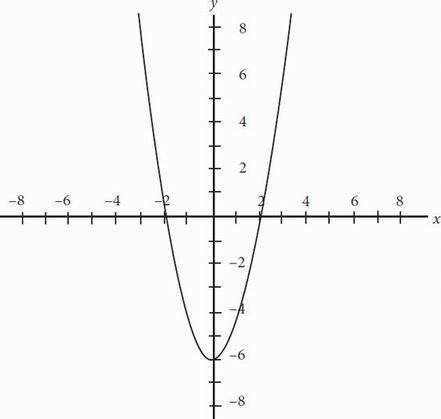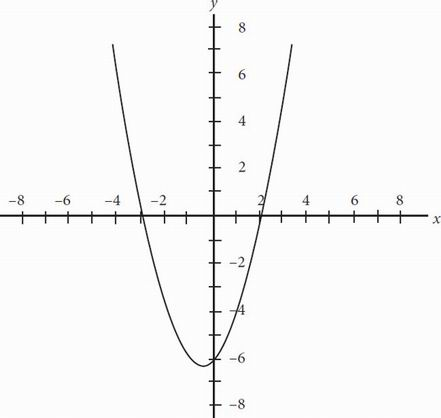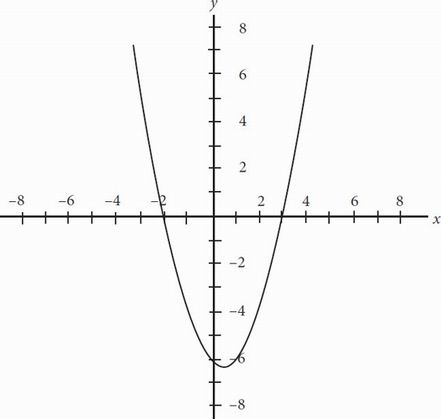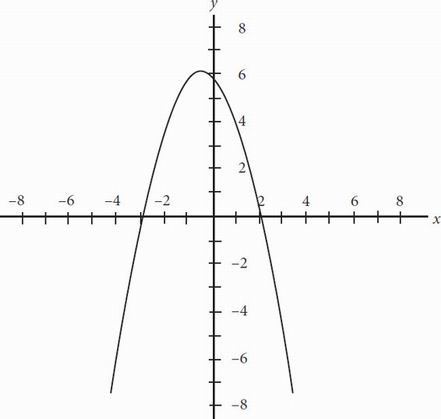# SAT Math Multiple Choice Question 147: Answer and Explanation

### Test Information

Question: 147

12. A certain polynomial, P, has a degree of 2. Polynomial P has zeros of 2 and -3, and a > 0 when the function of polynomial P is written in the form of y = ax2 + bx + c. Given this information, which of the following could be the graph of polynomial P ?

• A.• B.• C.• D.